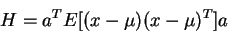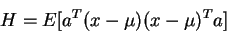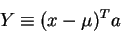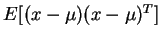# Appendix 2

In this note we prove that a covariance matrix is non-negative definite.

Consider the product, H, that is the product of an arbitrary vector, a, and the covariance vector, x:(1)

We can re-arrange the above by moving the constant vector a inside expectation operator so that we have,(2)

If we define(3)

(which is a random variable because x is), then (2) is

 H = E[ YT Y] (4)

This squared'' quantity is clearly never negative, so that we can conclude that the covariance matrixis non-negative definite.

Q.E.D

Skip Carter
1999-12-09